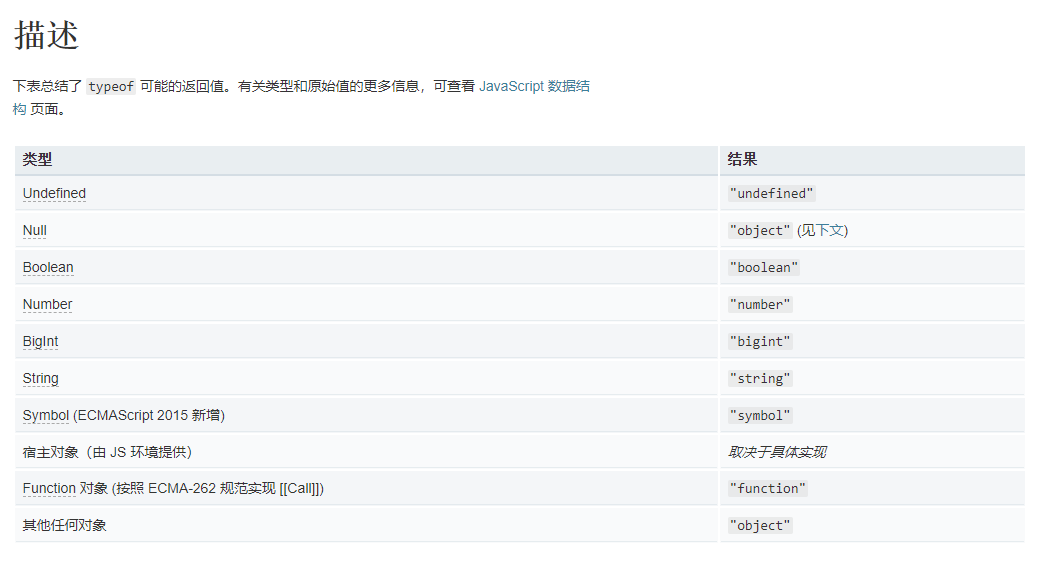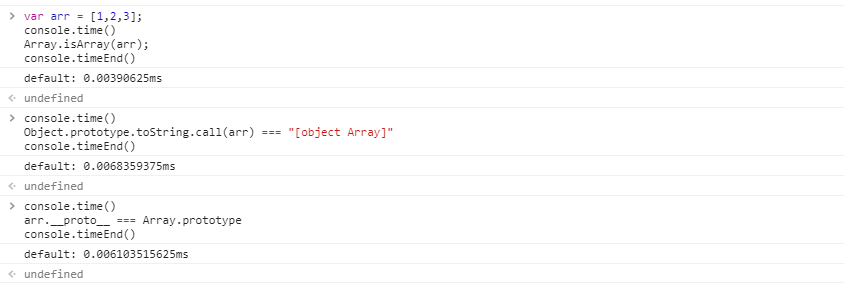# js判断一个对象是数组（函数）的方法实例_唇印_前端开发者

1.typeof操作符```// 数值
typeof 37 === 'number';

// 字符串
typeof '' === 'string';

// 布尔值
typeof true === 'boolean';

// Symbols
typeof Symbol() === 'symbol';

// Undefined
typeof undefined === 'undefined';

// 对象
typeof {a: 1} === 'object';
typeof [1, 2, 4] === 'object';

// 下面的例子令人迷惑，非常危险，没有用处。避免使用它们。
typeof new Boolean(true) === 'object';
typeof new Number(1) === 'object';
typeof new String('abc') === 'object';

// 函数
typeof function() {} === 'function';```

2.instanceof操作符 和 对象的constructor 属性

```var arr = [1,2,3,1];
console.log(arr instanceof Array); // true

var fun = function(){};
console.log(fun instanceof Function); // true```
```var arr = [1,2,3,1];
console.log(arr.constructor === Array); // true

var fun = function(){};
console.log(arr.constructor === Function); // true```

```var iframe = document.createElement('iframe'); //创建iframe
document.body.appendChild(iframe); //添加到body中
xArray = window.frames[window.frames.length-1].Array;
var arr = new xArray(1,2,3); // 声明数组[1,2,3]

4.使用 Object.prototype.toString 来判断是否是数组

```Object.prototype.toString.call( [] ) === '[object Array]' // true

Object.prototype.toString.call( function(){} ) === '[object Function]' // true```

5.使用 原型链 来完成判断

```[].__proto__ === Array.prototype // true

var fun = function(){}
fun.__proto__ === Function.prototype // true```

6.Array.isArray()

`Array.isArray([])  // true`

ECMAScript5将Array.isArray()正式引入JavaScript，目的就是准确地检测一个值是否为数组。IE9+、 Firefox 4+、Safari 5+、Opera 10.5+和Chrome都实现了这个方法。但是在IE8之前的版本是不支持的。

```var arr = [1,2,3];
var arr2 = [{ name : 'jack', age : 22 }];
function isArrayFn(value){
// 首先判断浏览器是否支持Array.isArray这个方法
if (typeof Array.isArray === "function") {
return Array.isArray(value);
}else{
return Object.prototype.toString.call(value) === "[object Array]";
// return obj.__proto__ === Array.prototype;
}
}
console.log(isArrayFn(arr)); // true
console.log(isArrayFn(arr2)); // true    ```

js如何判断一个对象是数组从

» 本文链接地址：https://www.rokub.com/71932.html
» 您也可以订阅本站：https://www.rokub.com
64K

• 回顶